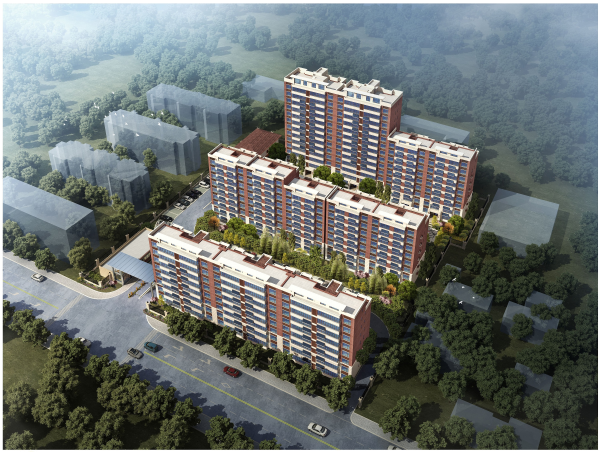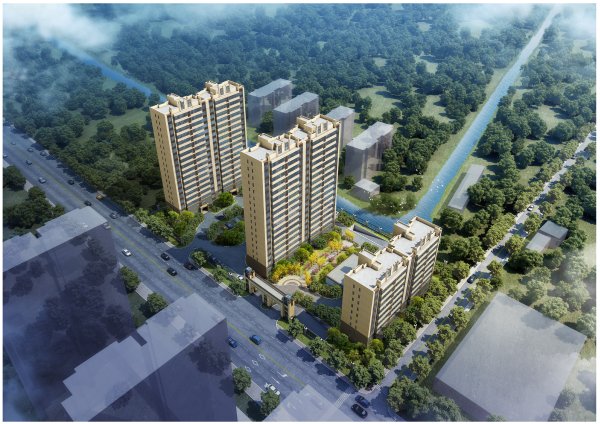## 网站地图## 鹤城碧桂园

### 南通

#### 地址

• 资阳
• 本溪
• 北流
• 万载
• 慈溪
• 长春
• 通化
• 西昌
• 丰城
• 阳春
• 鹤山
• 江油
• 辛集
• 辽源
• 衡水
• 黔西南州
• 黔东南州
• 石家庄
• 唐山
• 秦皇岛
• 邯郸
• 邢台
• 保定
• 张家口
• 承德
• 沧州
• 廊坊
• 黄骅
• 迁安
• 怀来
• 洛阳
• 驻马店
• 郑州
• 开封
• 平顶山
• 安阳
• 鹤壁
• 新乡
• 焦作
• 濮阳
• 许昌
• 漯河
• 三门峡
• 南阳
• 商丘
• 信阳
• 周口
• 武汉
• 黄石
• 十堰
• 宜昌
• 襄阳
• 荆州
• 荆门
• 鄂州
• 仙桃
• 潜江
• 黄冈
• 随州
• 咸宁
• 恩施
• 怀化
• 长沙
• 株洲
• 湘潭
• 湘西州
• 张家界
• 益阳
• 郴州
• 衡阳
• 岳阳
• 常德
• 娄底
• 徐州
• 南京
• 无锡
• 镇江
• 泰州
• 淮安
• 舟山
• 黔西南布依族苗族自治州
• 临沂
• 凉山
• 普洱
• 红河
• 锦州
• 葫芦岛
• 东方
• 咸阳
• 丽水
• 定安县
• 吕梁
• 来宾
• 河池
• 宜宾
• 重庆市
• 雅安
• 潮州
• 大理
• 巴中
• 桂林
• 贵港
• 百色
• 柳州
• 贺州
• 儋州
• 福清
• 威海
• 烟台
• 乐山
• 金华
• 永泰
• 潍坊
• 滨州
• 德州
• 临汾
• 日照
• 眉山
• 武威
• 乌鲁木齐
• 保山
• 临沧
• 防城港
• 达州
• 遂宁
• 绵阳
• 淮南
• 宝鸡
• 枣庄
• 桂平
• 景德镇
• 临夏
• 聊城
• 崇左
• 马鞍山
• 安顺
• 北海
• 菏泽
• 铜陵
• 黔南州
• 蚌埠
• 嘉兴
• 淮北
• 都匀
• 南宁
• 兴义
• 南平
• 松溪
• 吉安
• 大同
• 陆丰
• 厦门
• 铜仁
• 银川
• 昭通
• 包头
• 遵化
• 漳州
• 深圳
• 霸州
• 香港
• 合肥
• 抚州
• 琼海
• 定西
• 南昌
• 宿州
• 文昌
• 临高县
• 陵水
• 海口
• 博鳌
• 三亚
• 绥化
• 哈尔滨
• 杭州
• 绍兴
• 宁波
• 温州
• 衢州
• 湖州
• 台州
• 天津
• 沈阳
• 大连
• 通辽
• 兴安盟
• 呼伦贝尔
• 运城
• 晋中
• 太原
• 南通
• 宿迁
• 扬州
• 盐城
• 连云港
• 苏州
• 常州
• 安庆
• 黄山
• 巢湖
• 芜湖
• 池州
• 宣城
• 六安
• 古田
• 无为
• 桐城
• 亳州
• 南陵
• 庐江
• 阜阳
• 滁州
• 孝感
• 天门
• 邵阳
• 永州
• 平果
• 玉林
• 梧州
• 钦州
• 梅州
• 河源
• 云浮
• 东莞
• 汕头
• 中山
• 清远
• 惠州
• 韶关
• 肇庆
• 阳江
• 汕尾
• 茂名
• 江门
• 佛山
• 广州
• 雷州
• 英德
• 湛江
• 揭阳
• 珠海
• 信宜
• 济南
• 淄博
• 青岛
• 泰安
• 济宁
• 马来西亚雪兰莪州
• 马来西亚柔佛州
• 永春
• 南安
• 三明
• 宁德
• 武夷山
• 福鼎
• 莆田
• 建瓯
• 漳浦
• 福安
• 福州
• 泉州
• 龙岩
• 兰州
• 张掖
• 天水
• 白银
• 贵阳
• 仁怀
• 毕节
• 凯里
• 六盘水
• 遵义
• 广元
• 德阳
• 南充
• 泸州
• 攀枝花
• 广安
• 成都
• 宜春
• 萍乡
• 九江
• 赣州
• 上饶
• 曲靖
• 楚雄
• 昆明
• 白城
• 北京
• 澳洲莱德
• 西安
• 渭南
• 上海
• 西宁

27载匠心 给您一个五星级的家碧桂园 《财富》世界500强 中国地产三强

雄厚实力 品牌保障

碧桂园集团成立于1992年广东顺德，2007年4月港交所上市，是一家集地产、酒店、物业、教育、农业、科技于一体的综合性企业;27年始终坚持“给您一个五星级的家”的运营理念，参与全国超过1100个城镇打造超2000个高品质项目，服务超过400万业主家庭。持续带动中国城市现代化建设。

27载匠心 敬献千年鹤城

落子吕四 焕新一座城市

2019年4月，碧桂园启东第五子，首驻吕四，一举拿下吕四港镇陈涛路东侧、吕四港镇香堂路北侧两个地块。2公里范围内配套完善齐全，便享繁华生活;碧桂园将汲取27年建筑精髓，打造吕四改善居住的优选项目。

深耕南通 7载辉煌

筑就36子惊艳通城

自2012年，碧桂园首次进驻南通以来， 布局南通六县一市，成功打造36个精品项目，其中启东碧桂园大名府、启东蝶湖世界湾等城市作品，赢得广泛青睐;从布局南通的坚定步履，到生生不息的万家灯火。

鹤城路向东 为吕四焕新升级

鹤城碧桂园位于吕四商圈，周边商业配套醇熟，吃喝玩乐购娱全覆盖，项目2公里内，包含菜场、商业街、生活购物广场、人民广场等，满足未来生活的所有想象。

学校就在家门口 下楼即达的省心

鹤城中学、吕四中学环伺左右

项目周边学府林立，吕四港中心幼儿园、桂林小学、鹤城中学、吕四中学等，师资力量雄厚，教育资源优渥，12年全龄制的教育一应俱全。

全优社区配套 品质生活高阶定制

童梦乐园、有氧跑道领衔新人居

碧桂园立体园林景观，采用堆坡造景的设计手法，甄选多重景观植物，形成纷叠交错的立体式景观。配套童梦乐园，夜光跑道，光合有氧社区全龄配套生活。

上市物业 英国BSI认证

铂金物管 标配凤凰管家服务

碧桂园物业，从独创“铂金凤凰管家服务”而响彻全国，秉承着“给您一个五星级的家”的物业服务理念，为约 140 万户业主提供专业细致的社区服务，2019更是荣获中国社区服务商TOP1。

超400万业主 一致青睐共同选择

买的放心住的舒心 好房子一套就够了

作为碧桂园在吕四的首个重要作品，项目本着“初心、良心、匠心” 的品质追求，在安全、美观、经济、适用和耐久上反复推敲，充分借鉴启东、南通等标杆作品建筑精髓;建筑面积约91-124㎡现代美学洋房，敬献有成就的鹤城人。